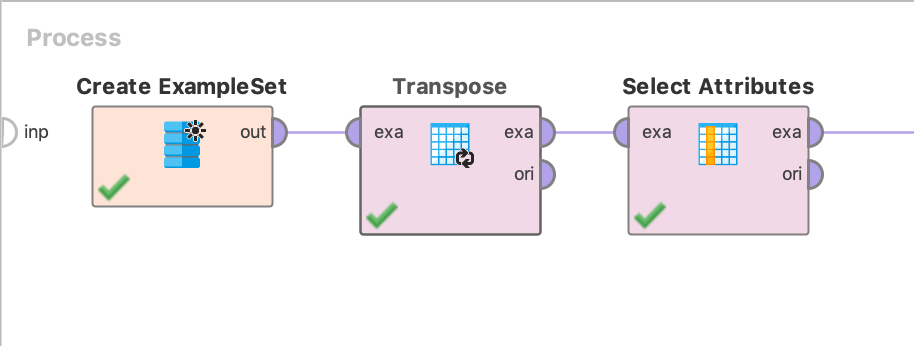# Write CSV of all attribute names from a lot of files

Member Posts: 1Newbie
edited June 2019 in Help
I'm trying to create a process for ingesting a lot of different excel files with varying formats and attribute names. I have created a small "attribute dictionary" that maps a number of different attribute names to the standardized versions that I need to move them to (i.e. Phone, phone, phone #, telephone, all get renamed to Phone). But there are 95+ different files that I need to account for (and hundreds more behind that) in order to ensure I have as many different formats covered as possible.

I have used the Loop Files operator and have a nice little macro and log subprocess in there but I can't figure out how to pass an entire set of attributes through to a csv especially when the number of attributes changes from one file to the next (lots of extraneous columns in some files).

How do I create a csv consisting of just the first row of each excel?
Tagged:

• Moderator, RapidMiner Certified Analyst, Member, University Professor Posts: 568Unicorn
Hello,

It's simple.
• First, Transpose the Excel file.
• Then, Select Attributes with the id.
• Finally, Write Excel or whatever you want to do.
Here is how it looks. Notice I am using "Create ExampleSet" because I am lazy and I don't want to generate an Excel file:Here is an example XML for you:
```<?xml version="1.0" encoding="UTF-8"?><process version="9.2.001">
<context>
<input/>
<output/>
<macros/>
</context>
<operator activated="true" class="process" compatibility="9.2.001" expanded="true" name="Process">
<parameter key="logverbosity" value="init"/>
<parameter key="random_seed" value="2001"/>
<parameter key="send_mail" value="never"/>
<parameter key="process_duration_for_mail" value="30"/>
<parameter key="encoding" value="SYSTEM"/>
<process expanded="true">
<operator activated="true" class="utility:create_exampleset" compatibility="9.2.001" expanded="true" height="68" name="Create ExampleSet" width="90" x="45" y="34">
<parameter key="generator_type" value="comma separated text"/>
<parameter key="number_of_examples" value="100"/>
<parameter key="use_stepsize" value="false"/>
<list key="function_descriptions"/>
<list key="numeric_series_configuration"/>
<list key="date_series_configuration"/>
<list key="date_series_configuration (interval)"/>
<parameter key="date_format" value="yyyy-MM-dd HH:mm:ss"/>
<parameter key="time_zone" value="SYSTEM"/>
<parameter key="column_separator" value=","/>
<parameter key="parse_all_as_nominal" value="false"/>
<parameter key="decimal_point_character" value="."/>
<parameter key="trim_attribute_names" value="true"/>
</operator>
<operator activated="true" class="transpose" compatibility="9.2.001" expanded="true" height="82" name="Transpose" width="90" x="179" y="34"/>
<operator activated="true" class="select_attributes" compatibility="9.2.001" expanded="true" height="82" name="Select Attributes" width="90" x="313" y="34">
<parameter key="attribute_filter_type" value="single"/>
<parameter key="attribute" value="id"/>
<parameter key="attributes" value=""/>
<parameter key="use_except_expression" value="false"/>
<parameter key="value_type" value="attribute_value"/>
<parameter key="use_value_type_exception" value="false"/>
<parameter key="except_value_type" value="time"/>
<parameter key="block_type" value="attribute_block"/>
<parameter key="use_block_type_exception" value="false"/>
<parameter key="except_block_type" value="value_matrix_row_start"/>
<parameter key="invert_selection" value="false"/>
<parameter key="include_special_attributes" value="false"/>
</operator>
<connect from_op="Create ExampleSet" from_port="output" to_op="Transpose" to_port="example set input"/>
<connect from_op="Transpose" from_port="example set output" to_op="Select Attributes" to_port="example set input"/>
<connect from_op="Select Attributes" from_port="example set output" to_port="result 1"/>
<portSpacing port="source_input 1" spacing="0"/>
<portSpacing port="sink_result 1" spacing="0"/>
<portSpacing port="sink_result 2" spacing="0"/>
</process>
</operator>
</process>
```
Hope it helps,

Rodrigo.